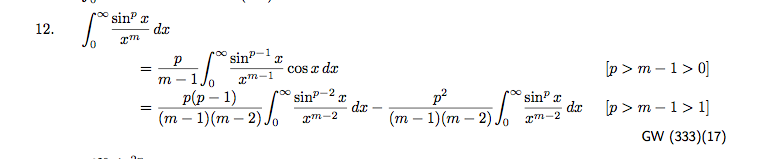# Can we simplify $\int_{0}^{\infty}\frac{{\sin}^px}{x^q}dx$?

We know the followings : $$\int_{0}^{\infty}\frac{{\sin}x}{x}dx=\int_{0}^{\infty}\frac{{\sin}^2x}{x^2}dx=\frac{\pi}{2},\int_{0}^{\infty}\frac{{\sin}^3x}{x^3}dx=\frac{3\pi}{8}.$$ Also, we can get $$\int_{0}^{\infty}\frac{{\sin}^3x}{x^2}dx=\frac{3\log 3}{4},\int_{0}^{\infty}\frac{{\sin}^4x}{x^3}dx=\log 2.$$ Then, I got interested in their generalization.

Question : Letting $p,q\in\mathbb N$, can we simplify the following? $$\int_{0}^{\infty}\frac{{\sin}^px}{x^q}dx$$

I don't have any good idea. Could you show me how to simplify this?

Remark : This question has been asked previously on math.SE without receiving any answers.

• math.stackexchange.com/questions/378547 You can extend the same technique to evaluate $$\int_{\mathbb{R}} \dfrac{\sin^m(x)}{x^n}dx$$ – user11000 Dec 2 '13 at 20:41

Gradshteyn and Ryzhik gives the recursion relation:(see also volume 1 of Integrals and Series by Prudnikov, Brychkov, and Marichev, page 387)

so if $$m$$ (your $$q$$) and $$p$$ are both even or both odd, with $$p\ge m$$ to assure convergence of the integral, we arrive at integrals of the form

$$\int_0^\infty \frac{\sin^{2n+1}x}{x}\,dx = \frac{(2n-1)!!}{(2n)!!}\frac{\pi}{2}$$

$$\int_0^\infty \frac{\sin^{2n}x}{x^2}\,dx = \frac{(2n-3)!!}{(2n-2)!!}\frac{\pi}{2}$$

This recursion produces the following closed form expression for $$p$$ and $$m$$ of the same parity (see Prudknikov, page 388):

$$\int_{0}^{\infty}\frac{\sin^{p}{x}}{x^{m}}dx=\frac{\pi}{2^{p}(m-1)!{\cal S}[(m-1)\pi/2]}$$ $$\qquad\qquad\times\sum_{j=0}^{[(p-1)/2]}(-1)^{[(p-1)/2]+j}\frac{p!(p-2j)^{(m-1)}}{j!(p-j)!}$$ with $${\cal S}(x)=\cos x$$ for $$p$$ odd and $${\cal S}(x)=\sin x$$ for $$p$$ even.

what remains is the case that $$m$$ and $$p$$ have different parity; the integral contains logarithms, but I haven't found a general formula (at least neither in Gradshteyn nor in Prudnikov).

• What if we would allow m to be any positive real number ? Or replace $\sin x$ with $|\sin x|$, and then allow p to be any positive real as well (with $m-p>1$, so as to ensure convergence) ? Perhaps the old formulas will still work, given that both simple and double factorials can be extended to non-natural arguments ? Or maybe not ? – Lucian Dec 3 '13 at 6:20
• @Lucian --- somehow I'm not able to get the logarithm (which I know should appear) by such a limiting procedure. – Carlo Beenakker Dec 3 '13 at 7:40

I'm posting an answer just to inform that the question has received an answer by Nick Strehlke on MSE.

\begin{align*} \int_0^\infty {\sin^p x\over x^q}\,dx & = \left\{\begin{array}{ll} \displaystyle{(-1)^{(p+q)/2}\pi\over 2^{p+1}(q-1)!}\sum_{k = 0}^p(-1)^k{p\choose k} |p - 2k|^{q-1} & \text{$p,q$ even,} \\[2em] \displaystyle {(-1)^{(p+q)/2-1}\pi\over 2^{p+1}(q-1)!}\sum_{k = 0}^p (-1)^k{p\choose k} \operatorname{sign}(p-2k) |p-2k|^{q-1} & \text{$p,q$ odd,} \\[2em] \displaystyle {(-1)^{(p+q+1)/2} \over 2^p (q-1)!} \sum_{k = 0\atop k\not = p/2}^p (-1)^k {p\choose k} |p-2k|^{q-1}\log{|p - 2k|} & \text{$p$ even, $q$ odd,} \\[2em] \displaystyle {(-1)^{(p+q-1)/2} \over 2^p (q-1)!} \sum_{k = 0\atop k\not = (p\pm1)/2}^p (-1)^k {p\choose k} \operatorname{sign}(p-2k) |p-2k|^{q-1}\log{|p - 2k|} & \text{$p$ odd, $q$ even,} \end{array}\right. \end{align*}

By the way, I noticed that these formulas can be simplified a bit as the followings which might be easier to calculate : $$\frac{(−1)^{(p+q+1)/2}}{2^{ p−q} (q−1)! } \sum_{k=0}^{ (p−4)/2} (−1)^k\binom pk\left(\frac p2−k\right)^{ q−1} \log\left(\frac p2−k\right)$$ for $p$ even and $q$ odd such that $3\le q\le p−1$ . $$\frac{(−1)^{(p+q-1)/2}}{2^{ p−1} (q−1)! } \sum_{k=0}^{ (p−3)/2} (−1)^k\binom pk\left(p−2k\right)^{ q−1} \log\left(p−2k\right)$$ for $p$ odd and $q$ even such that $2\le q\le p−1$ .

• This purpose of this comment is to make you aware that I see [Math Processing Error]s here. – Dirk Jan 15 '16 at 10:40

Suppose that $f$ is a smooth function which satisfies in the following condition

$$f(\pi+x)=f(x)\space \space and \space f(\pi-x)=f(x)$$ then, if the following integral exists

$$\int_0^\infty\frac{\sin^2x}{x^2}f(x)$$ We have the following nice equalities

$$\int_0^\infty\frac{\sin^2x}{x^2}f(x)=\int_0^{\infty}\frac{\sin x}{x}f(x)=\int_0^{\pi/2}f(x)dx$$

I proved the following formula as a part of my Bachelor project which extend the Lobachevsky's work that if we have $f(\pi+x)=f(x)\space \space and \space f(\pi-x)=f(x)$ then

$$\int_0^\infty\frac{\sin^4x}{x^4}f(x)=\int_0^{\pi/2}f(x)dx+\frac{2}{3}\int_0^{\pi/2}\sin^2 x\,f(x)dx$$

and I gave a method for finding an explicit formula for higher degree

$$\int_0^\infty\frac{\sin^{2n}x}{x^{2n}}f(x)$$

• How do you prove ? $$\int_0^\infty\frac{\sin^2x}{x^2}f(x)=\int_0^{\pi/2}\frac{\sin x}{x}f(x)=\int_0^{\pi/2}f(x)dx$$ – Duchamp Gérard H. E. Aug 19 '15 at 4:01
• Here is the proof, I am sorry for poor english of this paper, I wrote it in 2006 when I was third year of Bachelor degree arxiv.org/pdf/1004.2653.pdf – user21574 Aug 19 '15 at 4:56